Homework Help Question & Answers

# Part C 1 atm=760 mmHg=101,325 Pa What is the pressure (in atmospheres) of the gas inside...

Part C

1 atm=760 mmHg=101,325 Pa

What is the pressure (in atmospheres) of the gas inside the container connected to an open-end, mercury-filled manometer as shown in the picture? (Figure 1 shows 18 cm; cant attach photo) The atmospheric pressure is 0.95 atm. Express your answer numerically in atmospheres.

#### Homework Answers

Answer #1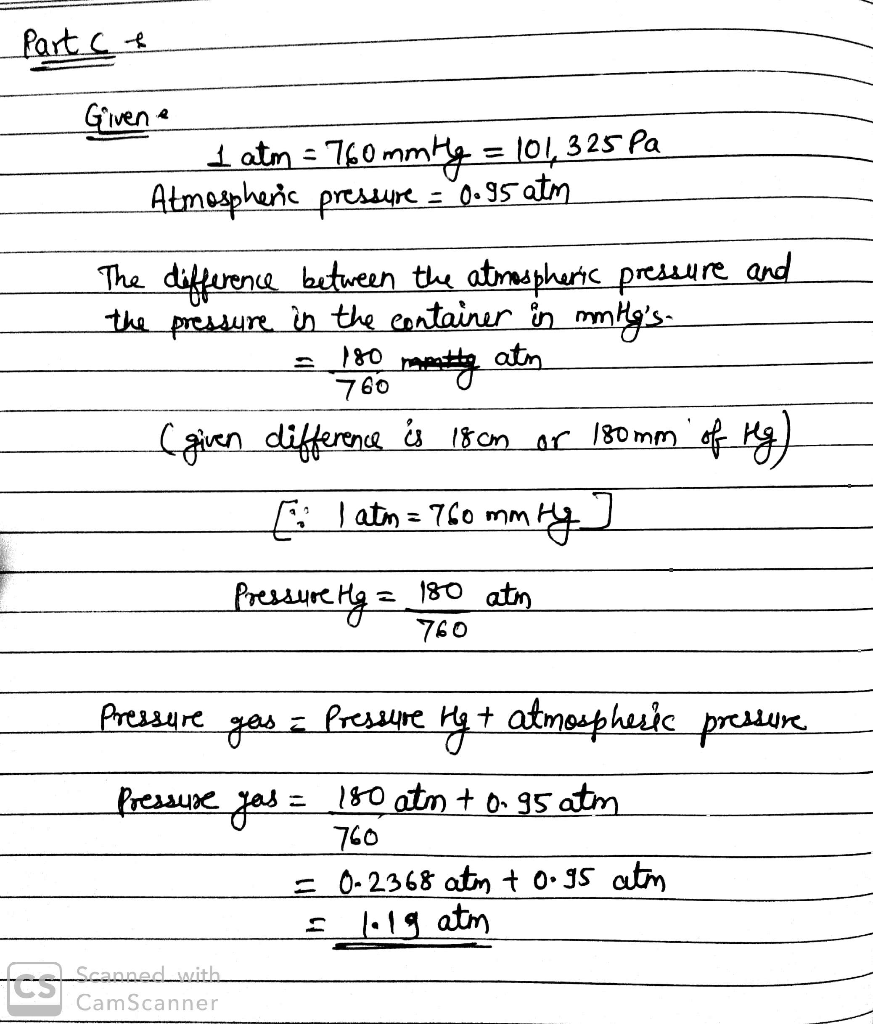Know the answer?
Your Answer:

#### Post as a guest

Your Name:

What's your source?

#### Earn Coin

Coins can be redeemed for fabulous gifts.

Not the answer you're looking for? Ask your own homework help question. Our experts will answer your question WITHIN MINUTES for Free.
Similar Homework Help Questions
• ### gas pressure

What is the pressure (in atmospheres) of the gas inside the container connected to an open-end, mercury-filled manometer as shown in the picture? The atmosphericpressure is 0.95 atm.Express your answer numerically in atmospheres.

• ### Gas pressure

1. A closed container is filled with oxygen. The pressure in thecontainer is 345 . What is the pressure in millimeters ofmercury?2. What is the pressure (in atmospheres) of the gas inside thecontainer connected to an open-end, mercury-filled manometer asshown in the picture?Theatmospheric pressure is 0.95.

• ### Gass pressure 3

What is the pressure (in atmospheres) of the gas inside the container connected to an open-end, mercury-filled manometer as shown in the picture? The atmosphericpressure is 0.95 .

• ### CHAPTER 5 Practice Problems Pressure Units 1 atm - 760 mmHg (exact) atm = 101325 Pa...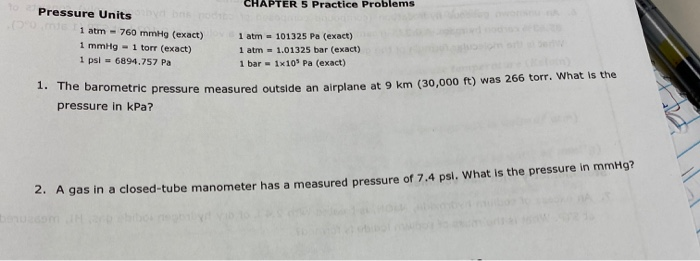CHAPTER 5 Practice Problems Pressure Units 1 atm - 760 mmHg (exact) atm = 101325 Pa (exact) 1 mmHg - 1 torr (exact) 1 atm = 1.01325 bar (exact) 1 psi = 6894.757 Pa 1 bar = 1x10 Pa (exact) 1. The barometric pressure measured outside an ometric pressure measured outside an airplane at 9 km (30,000 ft) was 266 torr. What is the pressure in kPa? 2. A gas in a closed-tube manometer has a measured pressure of 7.4...

• ### The liquid in the manometer is mercury. Determine the pressure of the gas in atm. Assume...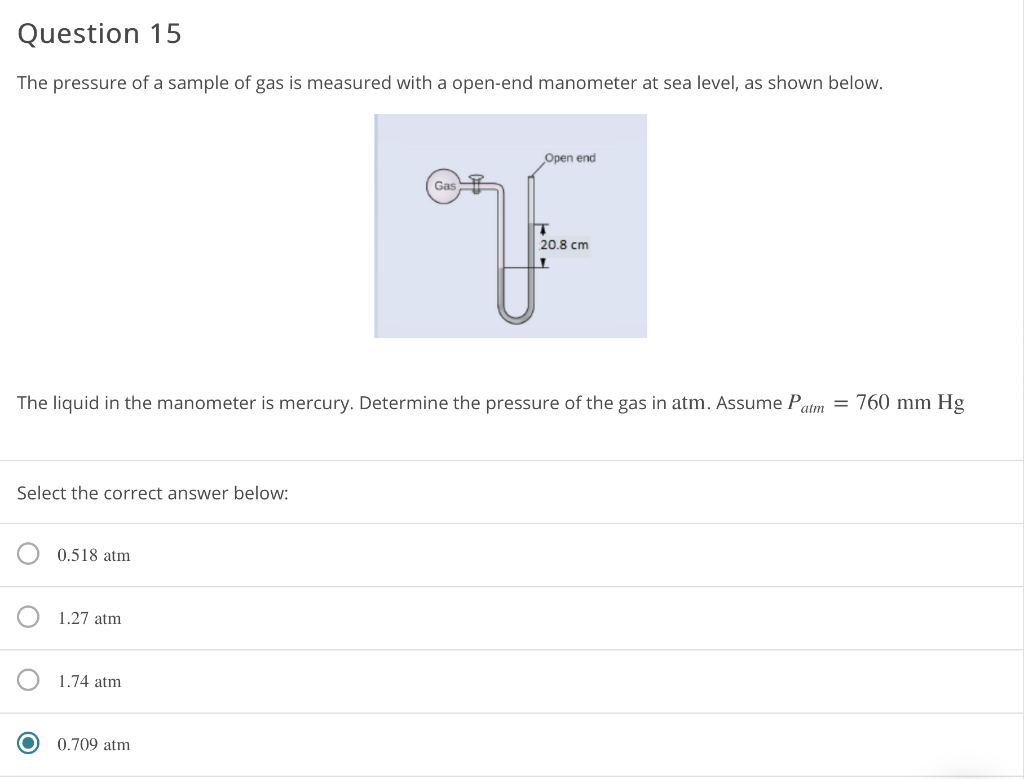The liquid in the manometer is mercury. Determine the pressure of the gas in atm. Assume Patm=760mm Hg Question 15 The pressure of a sample of gas is measured with a open-end manometer at sea level, as shown below. Open end Gas 20.8 cm The liquid in the manometer is mercury. Determine the pressure of the gas in atm. Assume Patm = 760 mm Hg Select the correct answer below: O 0.518 atm O 1.27 atm o 1.74 atm O...

• ### 12 of 33 Rewew Canstants Penodic Table has a pressure of 33.5 b/in. Comert 33.5 lb/in....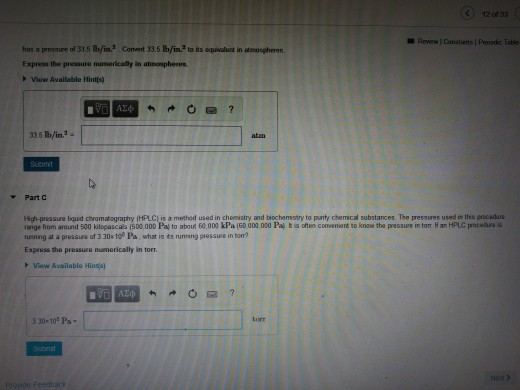12 of 33 Rewew Canstants Penodic Table has a pressure of 33.5 b/in. Comert 33.5 lb/in. to its equivalont in atmospheres Express the pressure numerically in atmospheres View Available Hintis) AE4 33.5 b/in2- atm Suomit Part C High-pressure liquid chromatography (HPLC) is a method used in chemistry and biochemistry to punty chemical substances. The pressures used in this procadure ange from around 500 kilopascals (500.00D Pal to about 60,000 kPa (60 0D0.000 Pal t is often comenient to know the...

• ### articular gas exerts a pressure of 731 mmHg. What is this pressure in units of atmospheres?...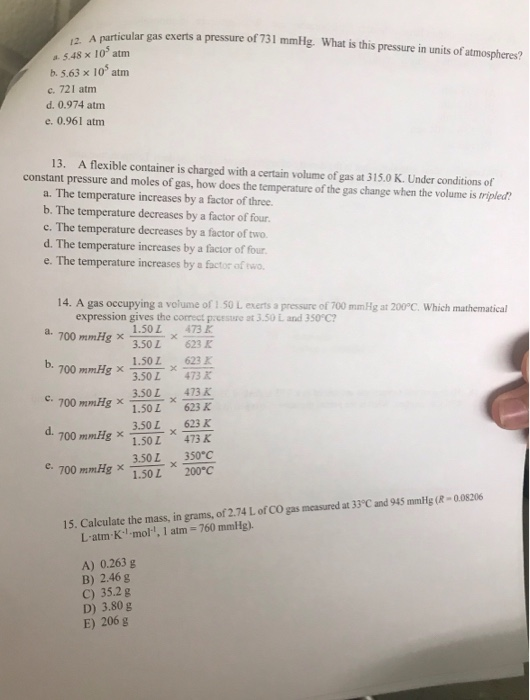articular gas exerts a pressure of 731 mmHg. What is this pressure in units of atmospheres? 12. A particular ga 5.48 x 10' atm b. 5.63 x 10 atm c. 721 atm d. 0.974 atm e. 0.961 atm 13. A flexible container is charged with a certain volume of gas at 315.0 K. Under conditions of constant pressure and moles of gas, how does the temperature of the gas change when the volume is tripled? a. The temperature increases by...

• ### At an atmospheric pressure of 745 mm Hg, what is the pressure of He gas inside a cylinder that is attached to an open-en...

At an atmospheric pressure of 745 mm Hg, what is the pressure of He gas inside a cylinder that is attached to an open-end manometer in which the level of mercury in the open side of the manometer is 25 mm Hg higher than the side that is attached to the gas cylinder?

• ### Part A The pressure inside a hydrogen-filled container was 2.10 atm at 21 ∘C. What would...

Part A The pressure inside a hydrogen-filled container was 2.10 atm at 21 ∘C. What would the pressure be if the container was heated to 99 ∘C ? Express your answer with the appropriate units. Part B At standard temperature and pressure (0 ∘C and 1.00 atm ), 1.00 mol of an ideal gas occupies a volume of 22.4 L. What volume would the same amount of gas occupy at the same pressure and 35 ∘C ? Express your answer...